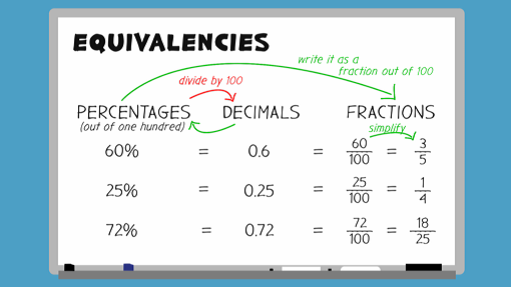Everyday maths 2 (Wales)

Start this free course now. Just create an account and sign in. Enrol and complete the course for a free statement of participation or digital badge if available.

Free course

# 9.1 Converting between percentages, decimals and fractions

Since fractions, decimals and percentages are all just different ways of representing the same thing, we can convert between them in order to compare. Take a look at the video below to see how to convert fractions, decimals and percentages.Skip transcript

#### Transcript

Equivalent fractions, decimals, and percentages are all just different ways of representing the same thing, so you can convert between them to compare. First, let's look at turning percentages into decimals. Take the example of 60%. Remember that a percentage is out of 100. To turn a percentage into its equivalent decimal, you need to divide by 100. 60 divided by 100 = 0.6. So 0.6 is the equivalent decimal of 60%.

What about 25%? Can you work out the equivalent decimal of 25% before the answer is revealed? Remember that 'cent' means 100.

To turn 25% into its equivalent decimal, you need to divide 25 by 100, which = 0.25.

Now let's find the equivalent fraction for the decimals we've calculated. To do this, you need to first turn it into a percentage and write it as a fraction out of 100. Using our previous examples, 0.6 or 60%, can be written as 60 over 100. 0.25, or 25%, can be written as 25 out of 100.

However, these fractions are not written in their simplest form. Remember that to simplify fractions, you need to divide both parts by the same number and keep going until you can't find a number that you can divide both parts by. In their simplest form, 0.6 is written as the fraction 3/5. 0.25 is written as the fraction one quarter, or 1 over 4.

Now looking at the table, you can see how percentages, decimals and fractions relate to each other.

Let's try one more example. Can you work out the equivalent decimal and fraction of 72%? 72 divided by 100 gives the decimal 0.72. To find the equivalent fraction, you need to change it into a percentage and then write it as a fraction out of 100. Then simplify the fraction into its simplest form, 18 out of 25, 18/25.

End transcript

Interactive feature not available in single page view (see it in standard view).

Lets look in more detail at changing a percentage to a fraction.

## Example: 50% is 50 divided by 100

As you can see this percentage is essentially a fraction of 100. However you can simplify it to .

To change a percentage to a fraction, put the percentage over 100 and simplify if possible.

Sometimes we might see a percentage like this: 12.5%.

If we use the method above we get but we can’t have a decimal in a fraction.

To get rid of the decimal in the fraction we must multiply the top and bottom of the fraction, the numerator and denominator, by any number that will give us whole numbers. In this case 10 or 2 both work well (12.5 × 10 = 125 and 12.5 × 2 = 25):

### Method 1: × 10

• × top and bottom by 10 = =

### Method 2: × 2

• × top and bottom by 2 = =

## Activity 21: Converting between percentages, decimals and fractions

1. Express these percentages as decimals:

• a.62%

• b.50%

• c.5%

2. Express these decimals as percentages:

• a.0.02

• b.0.2

• c.0.752

• d.0.055

3. Express these percentages as fractions:

• a.15%

• b.2.5%

• c.37.5%

1.

• a.0.62

• b.0.5

• c.0.05

2.

• a.2%

• b.20%

• c.75.2%

• d.5.5%

3.

• a. =

• b. × top and bottom by 10 = =

• c. × top and bottom by 10 = =

You may have multiplied by different numbers to get rid of the decimal in the last two questions. However, your final answers should still be the same as ours.

Now have a go at matching these fractions to decimals and percentages.

## Activity 22: Matching fractions, decimals and percentages

Choose the correct fraction for each percentage and decimal.

Using the following two lists, match each numbered item with the correct letter.

• a.      62.5% = 0.625 =

• b.      40% = 0.4 =

• c.      8% = 0.08 =

• d.      35% = 0.35 =

• 1 = d
• 2 = b
• 3 = c
• 4 = a

Next you’ll look in more detail at how to change a fraction to a percentage.

FSM_2_CYMRU#### Find out more

*Eligibility rules apply for financial support.